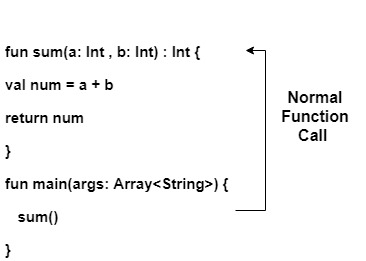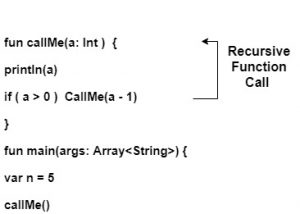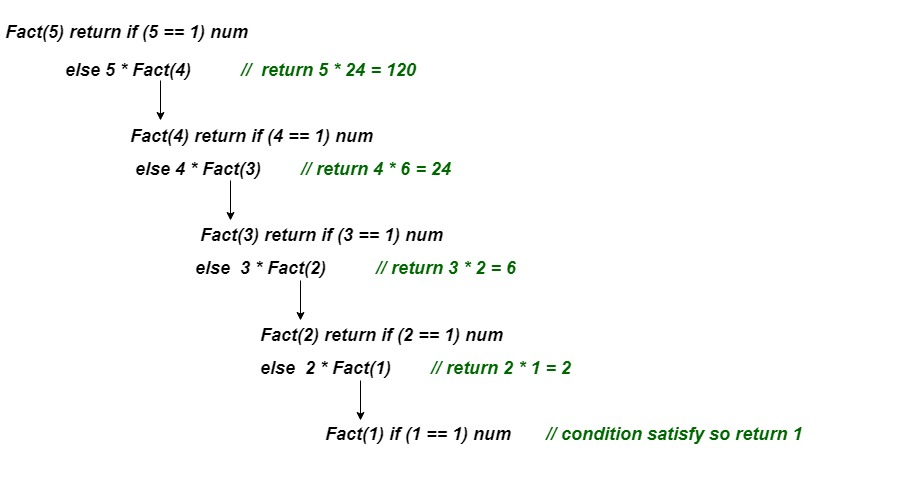# Kotlin Recursion

• Difficulty Level : Hard
• Last Updated : 12 Jun, 2019

In this tutorial we will learn Kotlin Recursive function. Like other programming languages, we can use recursion in Kotlin.
A function which calls itself is called as recursive function and this process of repetition is called recursion.

Whenever a function is called then there are two possibilities –

1. Normal function call
2. Recursive function call

## Normal function call

When a function is called from `main()` block then it is called a normal function call. In below example, `sum()` is called at a time and it executes its instruction and terminate with returning the sum of number. If we want to execute the function again then we should call `sum()` from the main block one more time.

Calling sum() function from main() block –## Recursive Function Call

When a function calls itself then it is called recursive function call. Every recursive function should have terminate condition else program executions enters in infinite loop and results into stack overflow error.

Calling callMe() function from its own block –Here, we have used terminate condition `if( a > 0)` else it enters the infinite loop. And it prints the value from 5 down to 0.

Example 1: Find the factorial of a number without using terminate condition

 `// Kotlin program of factorial using recursion``fun Fact(num: Int):Long{``    ``return`  `num*Fact(num-``1``)  ``// no terminate condition``}    ``//main method``fun main() {``    ``println(``"Factorial of 5 is: "``+Fact(``5``))``//Recursive call``}`

Output:

`Exception in thread "main" java.lang.StackOverflowError`

Example 2: Find the factorial of a number with using terminate condition.

 `// Kotlin program of factorial using recursion``fun Fact(num: Int):Long{``    ``return` `if``(num==``1``) num.toLong()        ``// terminate condition``    ``else` `num*Fact(num-``1``)``}``//main method``fun main() {``    ``println(``"Factorial of 5 is: "``+Fact(``5``))``//Recursive call``}`

Output:

`Factorial of 5 is: 120`

### Working of factorial program –

The recursive call of Fact() is explained step by step in the following figure:-Example 3: Find the sum of elements of an array using recursion

 `        ``// two parameters passed an array and size of array``fun sum(args: Array , index:Int ):Int{    ``    ``return` `if``(index<=``0``) ``0``    ``else` `(sum(args ,index-``1``) + args[index-``1``])      ``// recursive function call``}`` ` `fun main() {``    ``// array initialization``    ``val array = arrayOf(``1``,``2``,``3``,``4``,``5``,``6``,``7``,``8``,``9``,``10``)  ``    ``// size of array``    ``val n = array.size ``    ``val result = sum(array,n)             ``// normal function call``    ``println(``"The sum of array elements is: \$result"``)``}`

Output:

`The sum of array elements is: 55`

Explanation:
Here, we have initialized an array and passed as an argument to the sum() function. In each recursive call the index value decrement by one. If the index equal to zero or less than then terminate it and return the sum of all the elements.

My Personal Notes arrow_drop_up